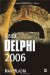Dynamic Arrays

All variables and arrays that we have used so far were static variables and static arrays. They are called static because their size is known at compile time, they are allocated on the stack, and they are automatically created and destroyed.

Dynamic arrays are different from static arrays in many ways:

• Dynamic arrays are manually allocated during run time.

• The first index in a dynamic array is always 0.

• Dynamic arrays are allocated on the heap.

The heap is the main memory area that is only limited by the amount of virtual memory available on the computer. If you need to hold a lot of data in an array, you should use a dynamic array because the size of static arrays is limited by the size of the stack.

The syntax for declaring a dynamic array is:

ArrayName: array of DataType;

When you declare a dynamic array, it contains no elements and no memory is allocated. To allocate space for the dynamic array, you have to call the SetLength function. SetLength accepts two parameters. When working with dynamic arrays, the first parameter is the dynamic array and the second parameter is an integer value that specifies the new size of the array.

Listing 6-7: Creating a dynamic arrayprogram Project1; {\$APPTYPE CONSOLE} uses   SysUtils; var   students: array of string;   stNumber: Integer;   cnt: Integer; begin   Write('Number of students: ');   ReadLn(stNumber);   SetLength(students, stNumber);   { Get student names from the user }   for cnt := Low(students) to High(students) do   begin     Write('Student: ');     ReadLn(students[cnt]);     if students[cnt] = 'end' then Break;   end;   ReadLn; end.To determine the size of the dynamic array, you can use the Length function, which accepts only one parameter — the dynamic array. The Length function returns the total number of elements in the dynamic array. The index of the last element is Length – 1, which is equal to High.

var   DynArray: array of string;   i: Integer; begin   for i := Low(DynArray) to High(DynArray) do ;   for i := 0 to Length(DynArray) - 1 do ;   ReadLn; end.

Both for loops execute the same number of times so it's up to you to decide which syntax you like best. If you want to be consistent, you should use the High and Low functions in loops like these and the Length function when you have to read the number of elements in the dynamic array. Note that both for loops are terminated with the semicolon. This means that the loops execute absolutely nothing. If your loop doesn't seem to function properly, make sure that you haven't added a semicolon after the reserved word do. Here is an illustration of this bug:

program Project1; {\$APPTYPE CONSOLE} uses   SysUtils; var   i: Integer; begin   for i := 1 to 2000 do ;   begin     WriteLn('This executes only once!');   end;   ReadLn; end.

Delphi also allows you to create dynamic multidimensional arrays. The syntax for declaring a dynamic multidimensional array is:

ArrayName: array of array of DataType;

The following example shows how to represent a chess table using a dynamic multidimensional array. All the pieces on the table are set to their default positions.

Listing 6-8: Working with a dynamic multidimensional arrayprogram Project1; {\$APPTYPE CONSOLE} uses   SysUtils; var   ChessTable: array of array of Integer;   i: Integer;   j: Integer; const   PAWN = 1;   ROOK = 2;   KNIGHT = 3;   BISHOP = 4;   QUEEN = 5;   KING = 6; begin   { allocate 8 empty arrays }   SetLength(ChessTable, 8);   for i := Low(ChessTable) to High(ChessTable) do   begin     { define length of every array }     SetLength(ChessTable[i], 8);     for j := Low(ChessTable[i]) to High(ChessTable[i]) do     begin       { set first line pieces }       if (i = 0) or (i = 7) then         case j of           0, 7: ChessTable[i, j] := ROOK;           1, 6: ChessTable[i, j] := KNIGHT;           2, 5: ChessTable[i, j] := BISHOP;           3: ChessTable[i, j] := QUEEN;           4: ChessTable[i, j] := KING;         end;       { set pawn line }       if (i = 1) or (i = 6) then         ChessTable[i, j] := PAWN;     end;      // for j   end;       // for i Finalize(ChessTable); end.Delphi automatically manages dynamic arrays. The memory allocated for a dynamic array is automatically deallocated when you no longer use the array. You can also manually deallocate the array by passing it to the Finalize procedure. The Finalize procedure deallocates the memory allocated for the ChessTable array.Inside Delphi 2006 (Wordware Delphi Developers Library)
ISBN: 1598220039
EAN: 2147483647
Year: 2004
Pages: 212